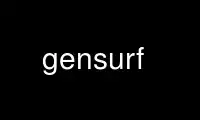# gensurf - Online in the CloudThis is the command gensurf that can be run in the OnWorks free hosting provider using one of our multiple free online workstations such as Ubuntu Online, Fedora Online, Windows online emulator or MAC OS online emulator

### PROGRAM:

#### NAME

gensurf - generate a RADIANCE or Wavefront description of a curved surface

#### SYNOPSIS

gensurf mat name 'x(s,t)' 'y(s,t)' 'z(s,t)' m n [ -e expr ][ -f file ][ -s ][ -o ]
gensurf mat name 'x(s,t)' 'y(s,t)' dfile m n [ -e expr ][ -f file ][ -s ][ -o ]
gensurf mat name dfile dfile dfile m n [ -s ][ -o ]

#### DESCRIPTION

Gensurf produces either a RADIANCE scene description or a Wavefront .OBJ file of a
functional surface defined by the parametric equations x(s,t), y(s,t), and z(s,t). The
surface normal is defined by the right hand rule as applied to (s,t). S will vary from 0
to 1 in steps of 1/m, and t will vary from 0 to 1 in steps of 1/n. The surface will be
composed of 2*m*n or fewer triangles and quadrilaterals. The expressions are of the same
type used in RADIANCE function files. Auxiliary expressions and/or files may be specified
in any number of -e and -f options. The -s option adds smoothing (surface normal
interpolation) to the surface. The -o option produces a Wavefront .OBJ file rather than a
RADIANCE scene description. This is most useful as input to the obj2mesh(1) program for
producing a compiled mesh. A single "usemtl" statement will appear at the beginning of
the .OBJ output, echoing the modifier given on the command line.

Rough holes may be cut in the mesh by defining a valid(s,t) function. Where this function
is positive, polygon vertices will be produced. Where it is negative, no geometry will be
output. Surface normal interpolation will ignore any invalid vertices.

The second invocation form reads z data values from the file dfile. This file must give
either m*n or (m+1)*(n+1) floating point z values. If m*n values are given, then the
values correspond to the centroid of each quadrilateral region. If (m+1)*(n+1) values are
given, then the values correspond to the vertices of each quadrilateral region. The
ordering of the data in the file is such that the s values are changing faster than the t
values. If a minus ('-') is given for dfile, then the values are read from the standard
input.

The third invocation form is used to read coordinate triplets from a file or the standard
input. The three dfile arguments must all be the same, and the corresponding file must
contain three floating point values for each point location. The ordering and other
details are the same as those described for z value files above.

#### EXAMPLE

To generate a tesselated sphere:

gensurf crystal ball 'sin(PI*s)*cos(2*PI*t)' 'cos(PI*s)' 'sin(PI*s)*sin(2*PI*t)' 7 10

To generate a 10x20 smoothed height field from 12 recorded vertex z values:

gensurf dirt ground '10*s' '20*t' height.dat 2 3 -s

Use gensurf online using onworks.net services

Free Servers & Workstations